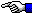# You have All the Money You Need

## Mathematical Proof that You already have All the Money You'll ever Need!

( Note: This page is intended to teach you something about Mathematics and reality. We do not actually believe in the truth of this proposition ! )

Let M be the amount of money you now have (your real cash assets), and let N be the amount of money you need. The average of these two amounts is given by: A = (M+N)/2. By applying simple algebra, we can also write this as 2A = M + N or

N + M = 2A.

Multiplying both sides of this equating by (N-M), we obtain:

(N + M) (N-M) = 2A (N-M)     (1)

or

N2 - M2 = 2AN - 2AM.    (2)

By transposing the M2 and the 2AN we have

N2 - 2AN = M2 - 2AM    (3)

and then add A2 to both sides to obtain:

N2 - 2AN + A2 = M2 - 2AM + A2.    (4)

Next, we factor both sides simplifying it to:

(N - A)2 = (M - A)2.    (5)

And taking the square root of both sides gives us just:

N - A = M - A,    (6)

or N = M (after adding A to both sides), thus the money you already have (M) is exactly what you need (N).

Assuming that you do not believe this proposition to be true ( if you do, please contact me in order to arrange a method whereby you can send some of your excess cash to people who still need some! ), then you owe it to yourself to figure out where and why an error exists in the calculations above!

If you want to know for sure whether or not you've discovered the errors in this so-called proof, then Read this page.The Starman's Math Index